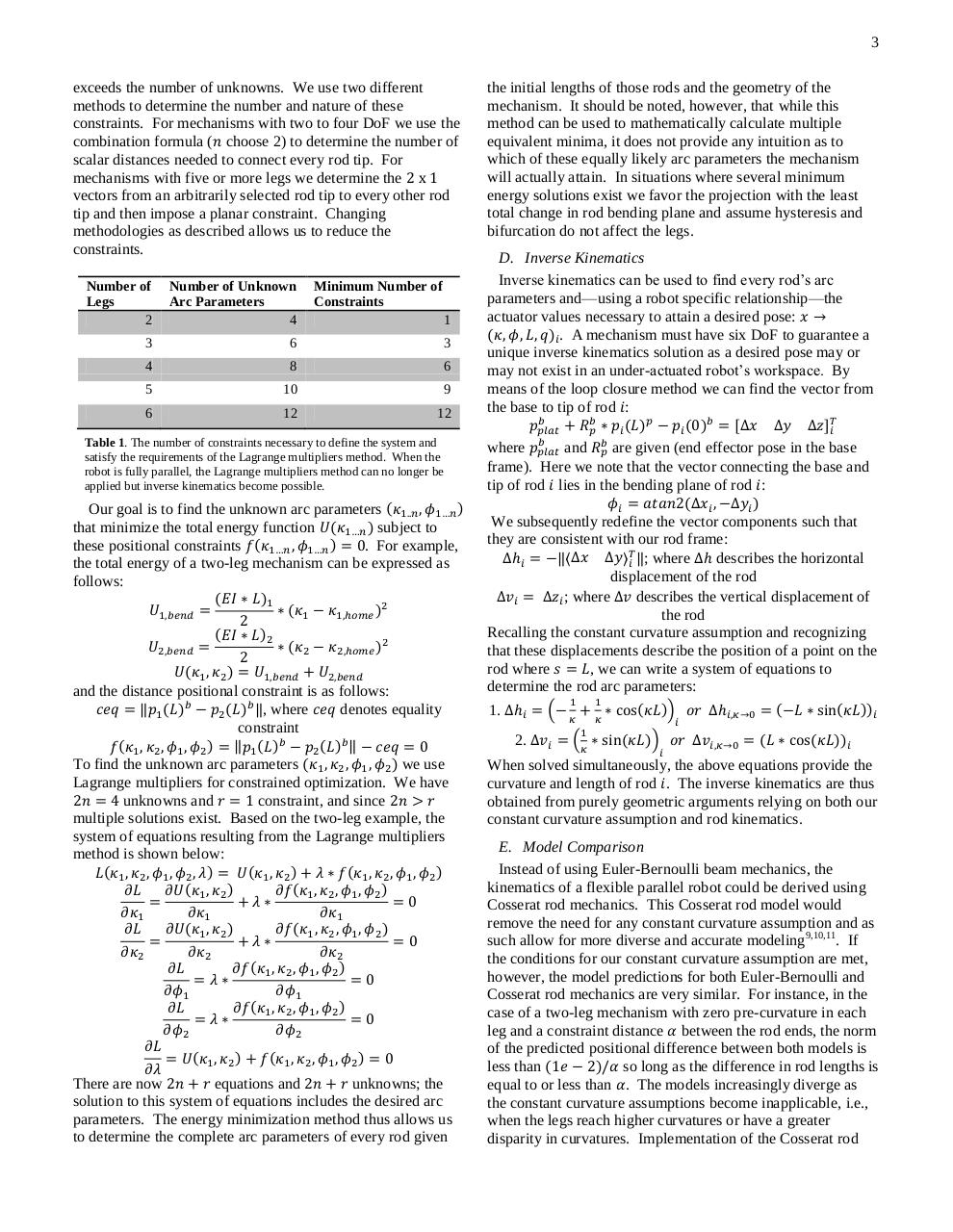# Flexible Parallel Robots, IEEE.pdfPage 1 2 3 4 5 6 7

#### Text preview

3
exceeds the number of unknowns. We use two different
methods to determine the number and nature of these
constraints. For mechanisms with two to four DoF we use the
combination formula ( choose 2) to determine the number of
scalar distances needed to connect every rod tip. For
mechanisms with five or more legs we determine the
vectors from an arbitrarily selected rod tip to every other rod
tip and then impose a planar constraint. Changing
methodologies as described allows us to reduce the
constraints.
Number of
Legs
2

Number of Unknown
Arc Parameters
4

Minimum Number of
Constraints

3

6

3

4

8

6

5

10

9

6

12

12

1

Table 1. The number of constraints necessary to define the system and
satisfy the requirements of the Lagrange multipliers method. When the
robot is fully parallel, the Lagrange multipliers method can no longer be
applied but inverse kinematics become possible.

Our goal is to find the unknown arc parameters
that minimize the total energy function
subject to
these positional constraints
. For example,
the total energy of a two-leg mechanism can be expressed as
follows:

and the distance positional constraint is as follows:

‖, where
denotes equality
constraint

To find the unknown arc parameters
we use
Lagrange multipliers for constrained optimization. We have
unknowns and
constraint, and since
multiple solutions exist. Based on the two-leg example, the
system of equations resulting from the Lagrange multipliers
method is shown below:

There are now
equations and
unknowns; the
solution to this system of equations includes the desired arc
parameters. The energy minimization method thus allows us
to determine the complete arc parameters of every rod given

the initial lengths of those rods and the geometry of the
mechanism. It should be noted, however, that while this
method can be used to mathematically calculate multiple
equivalent minima, it does not provide any intuition as to
which of these equally likely arc parameters the mechanism
will actually attain. In situations where several minimum
energy solutions exist we favor the projection with the least
total change in rod bending plane and assume hysteresis and
bifurcation do not affect the legs.
D. Inverse Kinematics
Inverse kinematics can be used to find every rod’s arc
parameters and—using a robot specific relationship—the
actuator values necessary to attain a desired pose:
. A mechanism must have six DoF to guarantee a
unique inverse kinematics solution as a desired pose may or
may not exist in an under-actuated robot’s workspace. By
means of the loop closure method we can find the vector from
the base to tip of rod :
[
]
where
and
are given (end effector pose in the base
frame). Here we note that the vector connecting the base and
tip of rod lies in the bending plane of rod :
We subsequently redefine the vector components such that
they are consistent with our rod frame:
‖〈
〉 ‖; where
describes the horizontal
displacement of the rod
; where
describes the vertical displacement of
the rod
Recalling the constant curvature assumption and recognizing
that these displacements describe the position of a point on the
rod where
, we can write a system of equations to
determine the rod arc parameters:
(

1.
2.

)
(

)

When solved simultaneously, the above equations provide the
curvature and length of rod . The inverse kinematics are thus
obtained from purely geometric arguments relying on both our
constant curvature assumption and rod kinematics.
E. Model Comparison
Instead of using Euler-Bernoulli beam mechanics, the
kinematics of a flexible parallel robot could be derived using
Cosserat rod mechanics. This Cosserat rod model would
remove the need for any constant curvature assumption and as
such allow for more diverse and accurate modeling9,10,11. If
the conditions for our constant curvature assumption are met,
however, the model predictions for both Euler-Bernoulli and
Cosserat rod mechanics are very similar. For instance, in the
case of a two-leg mechanism with zero pre-curvature in each
leg and a constraint distance between the rod ends, the norm
of the predicted positional difference between both models is
less than
so long as the difference in rod lengths is
equal to or less than . The models increasingly diverge as
the constant curvature assumptions become inapplicable, i.e.,
when the legs reach higher curvatures or have a greater
disparity in curvatures. Implementation of the Cosserat rod